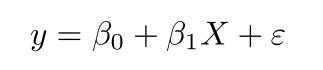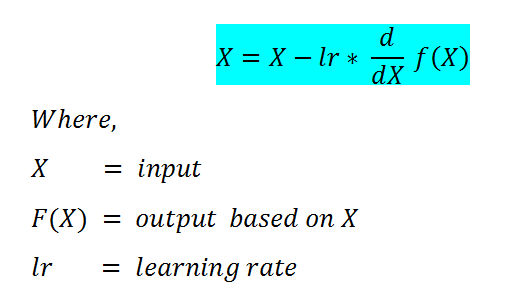Learn to Code via Tutorials on Repl.it!

← Back to all posts

# What's Linear Regression?

Linear Regression describes the relationship between variables by fitting a line to the observed data.

# Why do we need it?

We need it to predict house prices, from size and location (As you will see from the example).

# Why MLR?

MLR helps you predict between real data and predicted data using more than 2 variables.

# The equation:# The importing:

We need to import all the libraries that we need for the Linear Regression.

import matplotlib.pyplot as plt
import pandas as pd
from sklearn.model_selection import train_test_split
from sklearn.linear_model import LinearRegression
from sklearn.metrics import mean_squared_error

# The reading

Now we need to read the CSV file, my data is already cleaned up, but you will need to clean your data up, I'll cover this in a different tutorial.
For now, that's the code:

streeteasy = pd.read_csv("https://raw.githubusercontent.com/sonnynomnom/Codecademy-Machine-Learning-Fundamentals/master/StreetEasy/manhattan.csv")

df = pd.DataFrame(streeteasy)

x = df[['bedrooms', 'bathrooms', 'size_sqft', 'min_to_subway', 'floor', 'building_age_yrs', 'no_fee', 'has_roofdeck', 'has_washer_dryer', 'has_doorman', 'has_elevator', 'has_dishwasher', 'has_patio', 'has_gym']]

y = df[['rent']]

(We also declared x and y because they are two of our MLR variables).

# The train and test splitting for descent gradient

Gradient Descent is iterating between hundreds or even thousands of options and selecting the best one.
The selecting operation is done by an algorithm that scores each of the iterations by this formula:The learning rate is important, because sometimes it's too big, so the program takes hours to run, so you must decrease the learning rate, and sometimes you'll need to increase it.
The best score is 1, but it's impossible to reach it, because no algorithm is perfect.
That's the code:

x_train, x_test, y_train, y_test = train_test_split(x, y, train_size = 0.8, test_size = 0.2, random_state=6)

# Creating and fitting the model

Now, we just want to fit the model into SKlearn Linear Regression algorithm, and MathPlotLib plotting element so it will be clear, we'll also print the score of the x and y train.

lm = LinearRegression()

model = lm.fit(x_train, y_train)

y_predict= lm.predict(x_test)

print("Train score:")
print(lm.score(x_train, y_train))

print("Test score:")
print(lm.score(x_test, y_test))
plt.scatter(y_test, y_predict)

plt.plot(range(20000), range(20000))
plt.xlabel("Prices: $Y_i$")
plt.ylabel("Predicted prices: $\hat{Y}_i$")
plt.title("Actual Rent vs Predicted Rent")

plt.show()

Hope you enjoyed!
That's the full Repl:
MLR - Full Code

##### Comments
hotnewtop
ItamarCohen28 (10)

I really hoped that you enjoyed, it took me a long time and a free trial :)